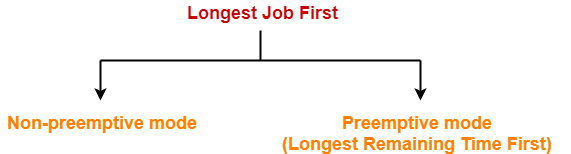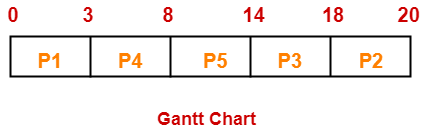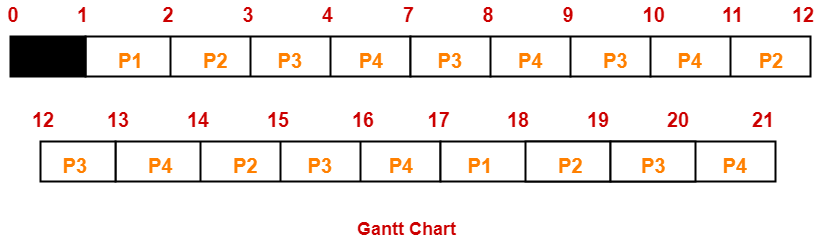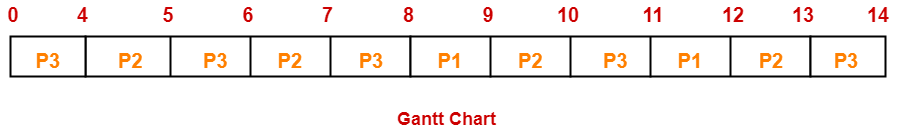## Longest Job First Algorithm-

In LJF Scheduling,

• Out of all the available processes, CPU is assigned to the process having largest burst time.
• In case of a tie, it is broken by FCFS Scheduling.• LJF Scheduling can be used in both preemptive and non-preemptive mode.
• Preemptive mode of Longest Job First is called as Longest Remaining Time First (LRTF).

• No process can complete until the longest job also reaches its completion.
• All the processes approximately finishes at the same time.

• The waiting time is high.
• Processes with smaller burst time may starve for CPU.

## Problem-01:

Consider the set of 5 processes whose arrival time and burst time are given below-

 Process Id Arrival time Burst time P1 0 3 P2 1 2 P3 2 4 P4 3 5 P5 4 6

If the CPU scheduling policy is LJF non-preemptive, calculate the average waiting time and average turn around time.

## Solution-

### Gantt Chart-Now, we know-

• Turn Around time = Exit time – Arrival time
• Waiting time = Turn Around time – Burst time

Also read- Various Times of Process

 Process Id Exit time Turn Around time Waiting time P1 3 3 – 0 = 3 3 – 3 = 0 P2 20 20 – 1 = 19 19 – 2 = 17 P3 18 18 – 2 = 16 16 – 4 = 12 P4 8 8 – 3 = 5 5 – 5 = 0 P5 14 14 – 4 = 10 10 – 6 = 4

Now,

• Average Turn Around time = (3 + 19 + 16 + 5 + 10) / 5 = 53 / 5 = 10.6 unit
• Average waiting time = (0 + 17 + 12 + 0 + 4) / 5 = 33 / 5 = 6.6 unit

## Problem-02:

Consider the set of 4 processes whose arrival time and burst time are given below-

 Process Id Arrival time Burst time P1 1 2 P2 2 4 P3 3 6 P4 4 8

If the CPU scheduling policy is LJF preemptive, calculate the average waiting time and average turn around time.

## Solution-

### Gantt Chart-Now, we know-

• Turn Around time = Exit time – Arrival time
• Waiting time = Turn Around time – Burst time

 Process Id Exit time Turn Around time Waiting time P1 18 18 – 1 = 17 17 – 2 = 15 P2 19 19 – 2 = 17 17 – 4 = 13 P3 20 20 – 3 = 17 17 – 6 = 11 P4 21 21 – 4 = 17 17 – 8 = 9

Now,

• Average Turn Around time = (17 + 17 + 17 + 17) / 4 = 68 / 4 = 17 unit
• Average waiting time = (15 + 13 + 11 + 9) / 4 = 48 / 4 = 12 unit

## Problem-03:

Consider three processes (process id 0, 1, 2 respectively) with compute time bursts 2, 4 and 8 time units. All processes arrive at time zero. Consider the longest remaining time first (LRTF) scheduling algorithm. In LRTF, ties are broken by giving priority to the process with the lowest process id. The average turn around time is-

1. 13 unit
2. 14 unit
3. 15 unit
4. 16 unit

## Solution-

We have the set of 3 processes whose arrival time and burst time are given below-

 Process Id Arrival time Burst time P1 0 2 P2 0 4 P3 0 8

### Gantt Chart-Now, we know-

Turn Around time = Exit time – Arrival time

 Process Id Exit time Turn Around time P1 12 12 – 0 = 12 P2 13 13 – 0 = 13 P3 24 14 – 0 = 14

Now,

Average Turn Around time = (12 + 13 + 14) / 3 = 39 / 3 = 13 unit

Thus, Option (A) is correct.

To gain better understanding about LJF Scheduling,

Watch this Video Lecture

To gain better understanding about LRTF Scheduling,

Watch this Video Lecture

Next Article- Highest Response Ratio Next Scheduling

Get more notes and other study material of Operating System.

Watch video lectures by visiting our YouTube channel LearnVidFun.